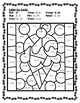# Summer/End of the Year Multiplication WorksheetsSubject
Resource Type
File Type

PDF

(17 MB|23 pages)
Product Rating
4.0
(2 Ratings)
Standards
Also included in:
1. Easy to print and fun for your students to practice their multiplication skill. Use them in math centers, independent practice, and Homework. Each product included has at least 10 worksheets that focus on multiplication skills. Skills from fast facts to multiplying multi-digit problems to word probl
\$24.00
\$12.00
Save \$12.00
• Product Description
• StandardsNEW

Summer Themed Multiplication Printable Worksheets are in black and white. An answer key is included. 10 printable worksheets included. Fast Facts, Missing numbers, Multiplying 3 digit by 1 digit. Multiplying 3 digit by 3 digit, Multiplying 3 digit by 2 digit, multiplication word problems (one-step).

This product is included in Holiday/Seasonal Multiplication Worksheets. If you have purchased this bundle, simply go to my purchases and download the newly added resource.

Looking for more Spring Math Activities:

End of the Year Math Scavenger Hunt

More End of the Year/ Summer Products

Fluently multiply and divide within 100, using strategies such as the relationship between multiplication and division (e.g., knowing that 8 × 5 = 40, one knows 40 ÷ 5 = 8) or properties of operations. By the end of Grade 3, know from memory all products of two one-digit numbers.
Determine the unknown whole number in a multiplication or division equation relating three whole numbers. For example, determine the unknown number that makes the equation true in each of the equations 8 × ? = 48, 5 = __ ÷ 3, 6 × 6 = ?.
Use multiplication and division within 100 to solve word problems in situations involving equal groups, arrays, and measurement quantities, e.g., by using drawings and equations with a symbol for the unknown number to represent the problem.
Interpret products of whole numbers, e.g., interpret 5 × 7 as the total number of objects in 5 groups of 7 objects each. For example, describe a context in which a total number of objects can be expressed as 5 × 7.
Multiply a whole number of up to four digits by a one-digit whole number, and multiply two two-digit numbers, using strategies based on place value and the properties of operations. Illustrate and explain the calculation by using equations, rectangular arrays, and/or area models.
Total Pages
23 pages
Included
Teaching Duration
N/A
Report this Resource to TpT
Reported resources will be reviewed by our team. Report this resource to let us know if this resource violates TpT’s content guidelines.